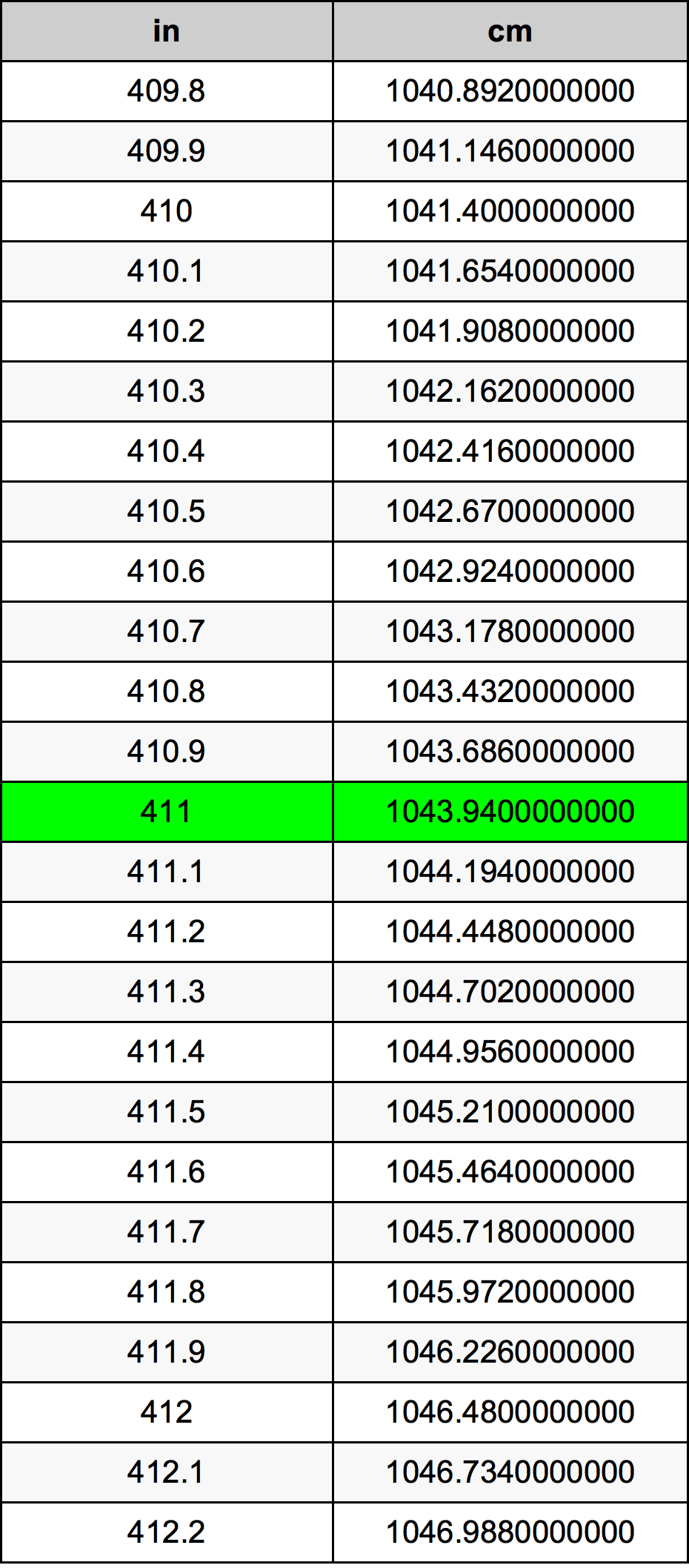Inches To Centimeters

# 411 in to cm411 Inches to Centimeters

in
=
cm

## How to convert 411 inches to centimeters?

 411 in * 2.54 cm = 1043.94 cm 1 in
A common question is How many inch in 411 centimeter? And the answer is 161.811023622 in in 411 cm. Likewise the question how many centimeter in 411 inch has the answer of 1043.94 cm in 411 in.

## How much are 411 inches in centimeters?

411 inches equal 1043.94 centimeters (411in = 1043.94cm). Converting 411 in to cm is easy. Simply use our calculator above, or apply the formula to change the length 411 in to cm.

## Convert 411 in to common lengths

UnitUnit of length
Nanometer10439400000.0 nm
Micrometer10439400.0 µm
Millimeter10439.4 mm
Centimeter1043.94 cm
Inch411.0 in
Foot34.25 ft
Yard11.4166666667 yd
Meter10.4394 m
Kilometer0.0104394 km
Mile0.0064867424 mi
Nautical mile0.0056368251 nmi

## What is 411 inches in cm?

To convert 411 in to cm multiply the length in inches by 2.54. The 411 in in cm formula is [cm] = 411 * 2.54. Thus, for 411 inches in centimeter we get 1043.94 cm.

## 411 Inch Conversion Table## Alternative spelling

411 Inches to cm, 411 Inches in cm, 411 Inch to Centimeter, 411 Inch in Centimeter, 411 in to Centimeters, 411 in in Centimeters, 411 Inches to Centimeter, 411 Inches in Centimeter, 411 in to Centimeter, 411 in in Centimeter, 411 Inch to cm, 411 Inch in cm, 411 Inches to Centimeters, 411 Inches in Centimeters